Updated date:

# Calculator Techniques for Circles and Triangles in Plane Geometry

Author:

Ray is a licensed engineer in the Philippines. He loves to write about mathematics and civil engineering.

## Inscribed and Circumscribed Circles

A circle can either be inscribed or circumscribed. A circle circumscribing a triangle passes through the vertices of the triangle while a circle inscribed in a triangle is tangent to the three sides of the triangle. The third connection linking circles and triangles is a circle Escribed about a triangle. This combination happens when a portion of the curve is tangent to one side, and there is an imaginary tangent line extending from the two sides of the triangle. Given A, B, and C as the sides of the triangle and A as the area, the formula for the radius of a circle circumscribing a triangle is r = ABC / 4A and for a circle inscribed in a triangle is r = A / S where S = (A + B + C) / 2.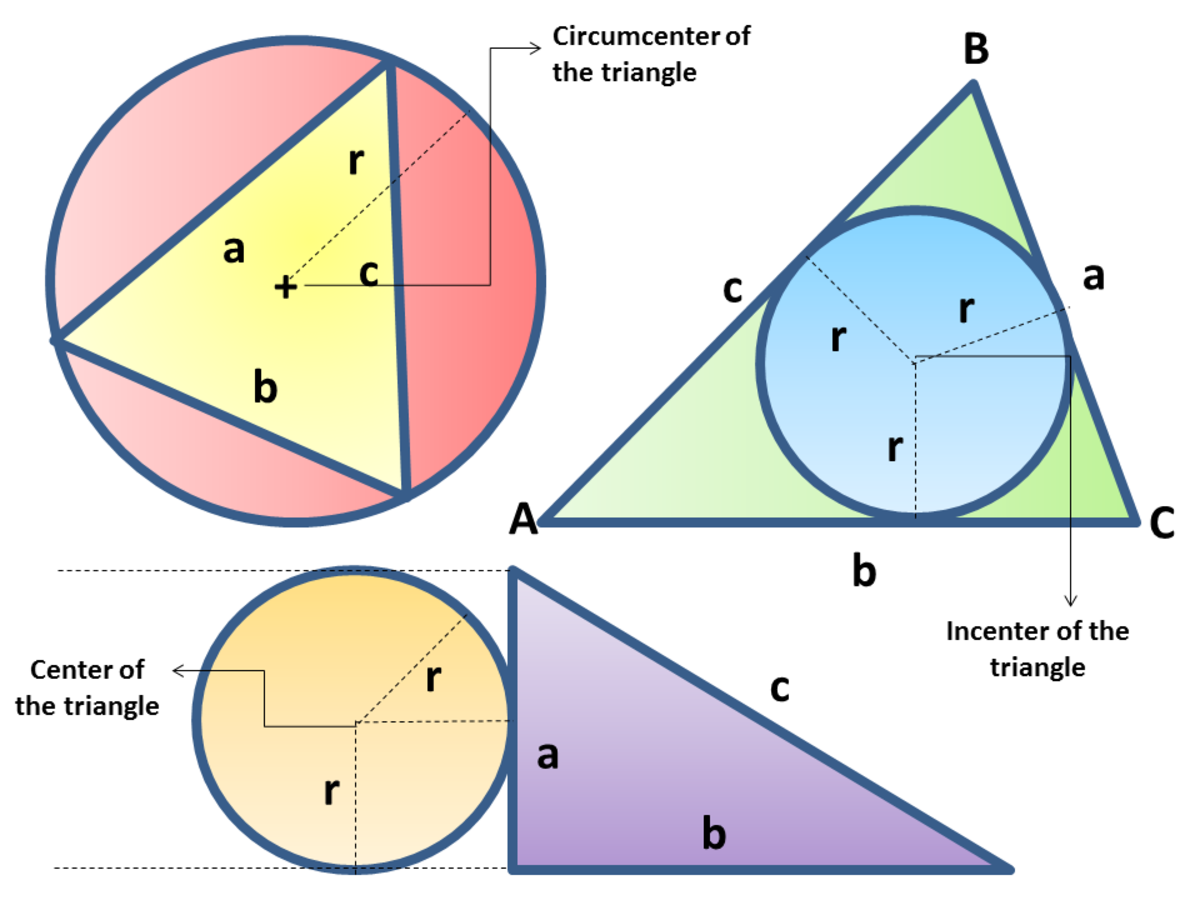Calculator Techniques for Circles and Triangles in Plane GeometryJohn Ray Cuevas

## Calculator Techniques for Circles and Triangles

Calculator techniques for problems related to circles and triangles are more on algebra, trigonometry, and geometry. Memorization of formulas is what is needed. Here are the contents of the article.

• A circle inscribed in a triangle
• Circle circumscribing a triangle
• Three circles mutually tangent to each other
• Area of a sector
• A circle inscribed in a sector
• The bisector of a triangle
• The side measures of a triangle
• Chord of a circle
• A point outside a triangle

### Problem 1: Circle Inscribed in a Triangle

The sides of a triangle are 8 cm, 10 cm, and 14 cm. Determine the radius of the inscribed circle.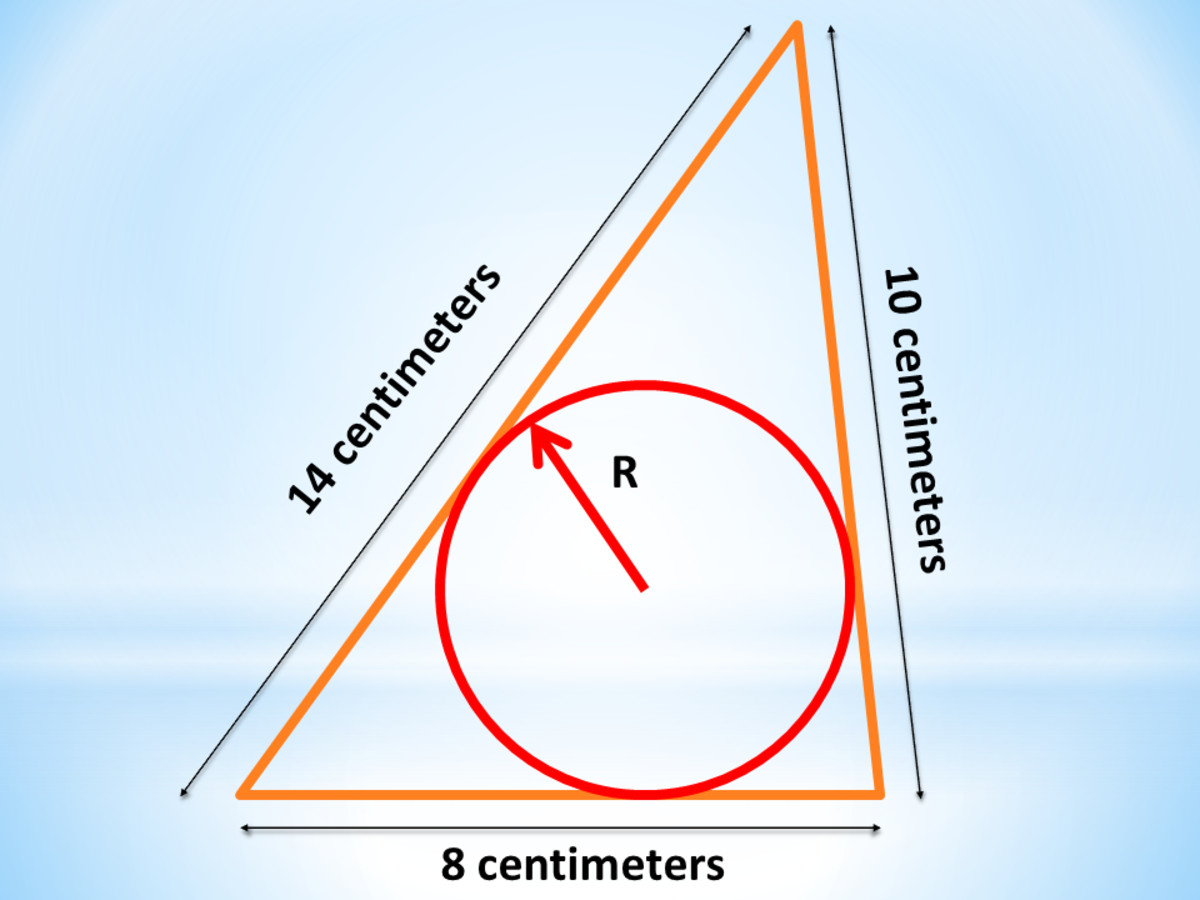Circle Inscribed in a Triangle: Calculator Techniques for Circles and Triangles in Plane GeometryJohn Ray Cuevas

Calculator Technique

a. Using Heron's formula, solve for the area of the triangle.

b. Solve for the perimeter of the triangle.

c. Solve for the radius of the inscribed circle.

### Problem 2: Circle Circumscribing an Equilateral Triangle

The area of a circle circumscribing about an equilateral triangle is 250.45 square meters. What is the area of the triangle?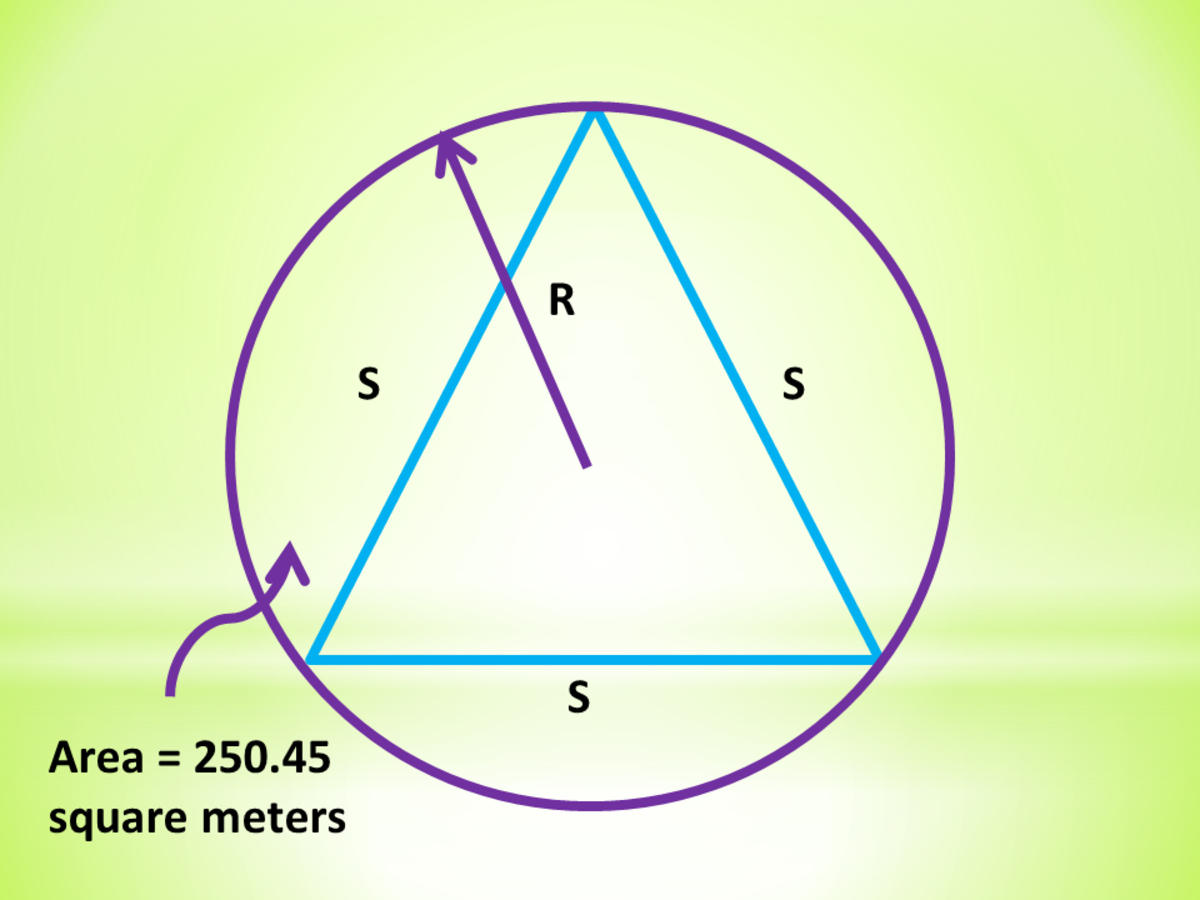Circle Circumscribing an Equilateral Triangle: Calculator Techniques for Circles and Triangles in Plane GeometryJohn Ray Cuevas

Calculator Technique

a. Given the area of the circle, solve for the radius.

b. Solve for a side of the equilateral triangle.

c. Solve for the area of the equilateral triangle.

Final Answer: The area of the equilateral triangle inscribed in a circle is 103.59 square meters.

### Problem 3: Three Circles Mutually Tangent

The distance between the centers of the three circles which are mutually tangent to each other externally is 10, 12 and 14 units. What is the area of the largest circle?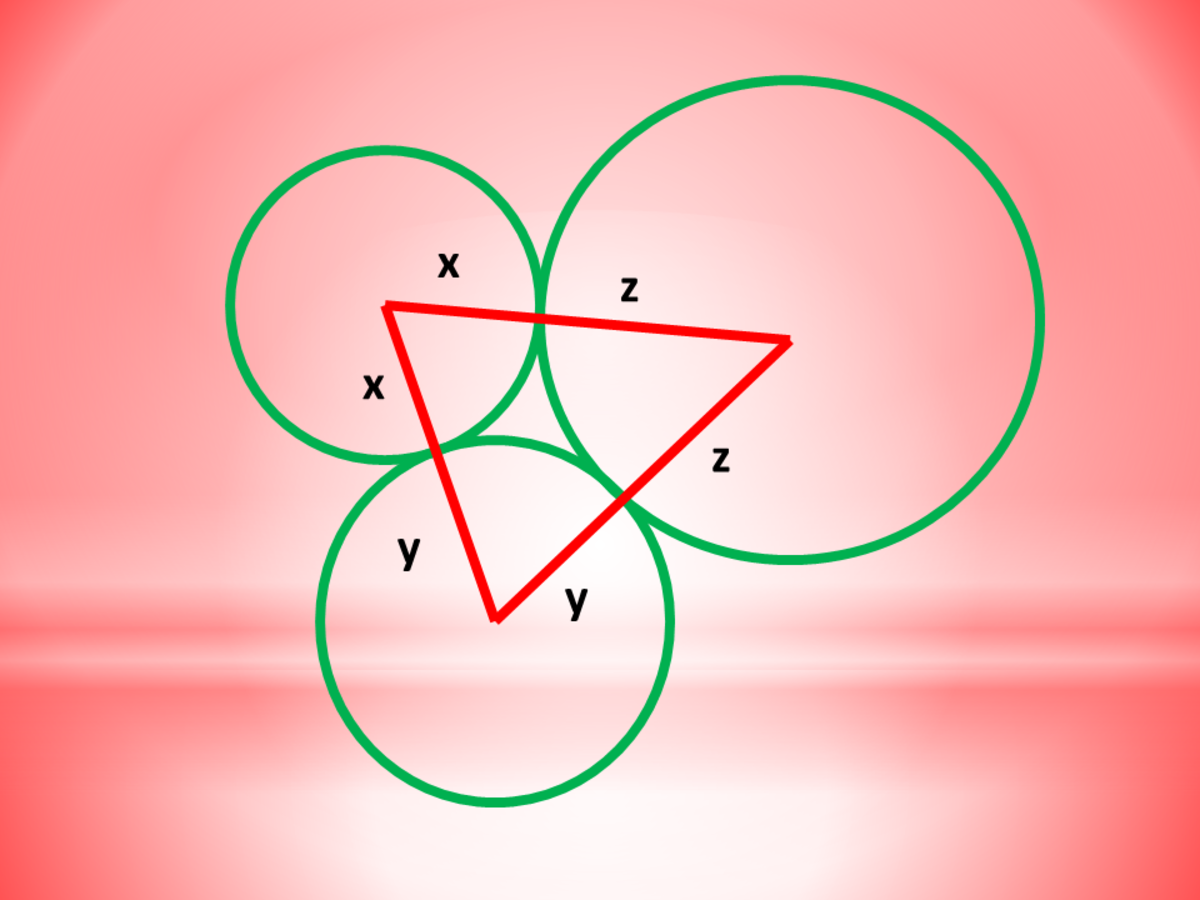Three Circles Mutually Tangent: Calculator Techniques for Circles and Triangles in Plane GeometryJohn Ray Cuevas

Calculator Technique

a. Form three equations for three unknowns.

b. Arrange the equations in a system form.

c. Solve the area of the largest circle using the largest radius from step 2.

Final Answer: The area of the largest circle is 201.06 square units.

### Problem 4: Triangle Inscribed in a Circle

The area of the triangle inscribed in a circle is 39.19 square centimeters, and the radius of the circumscribed circle is 7.14 centimeters. If the two sides of the inscribed triangle are 8 centimeters and 10 centimeters respectively, find the 3rd side.

Calculator Technique

a. Solve for the third side C.

Final Answer: The length of the third side is 14.00 centimeters.

### Problem 5: Area of a Sector

The angle of a circle's sector is 300 degrees, and the radius is 15 centimeters. Find the area in square centimeters.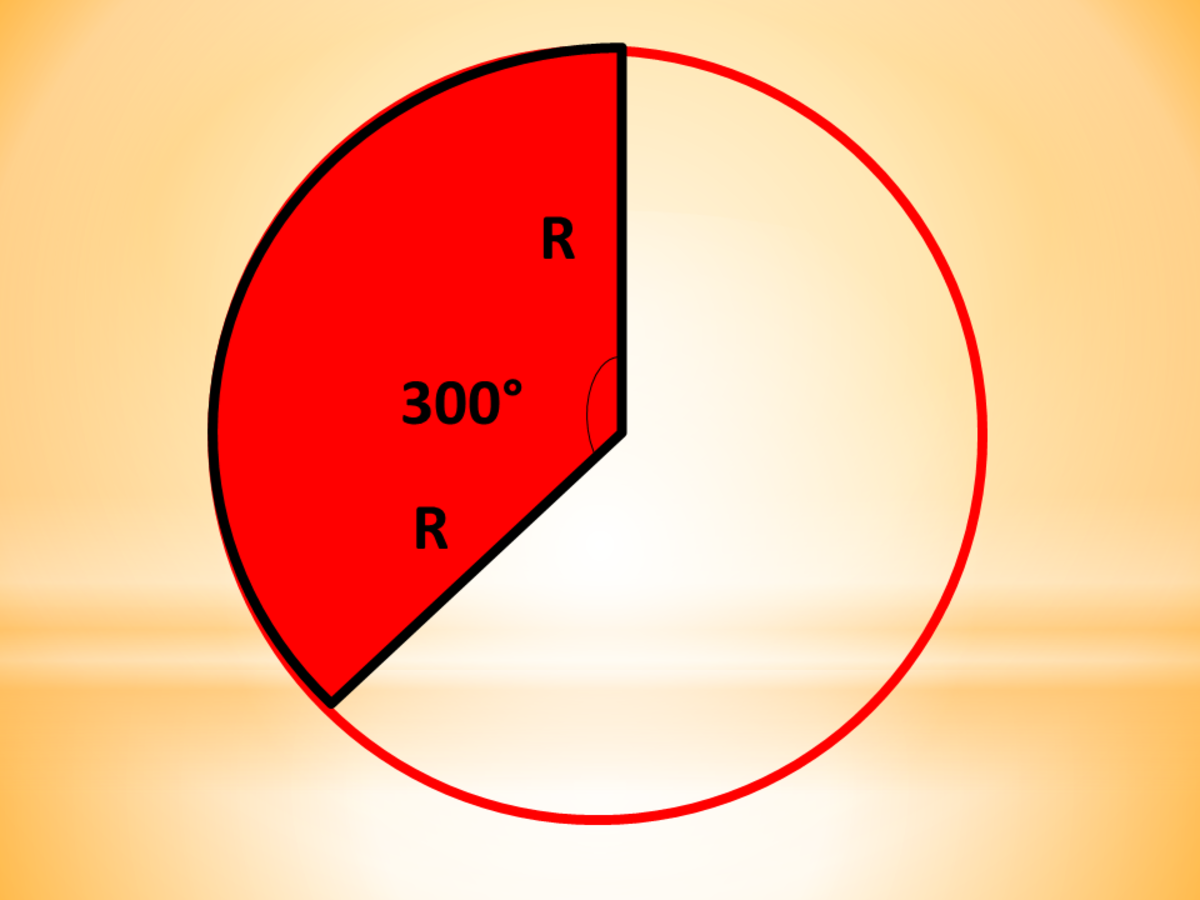Area of a Sector: Calculator Techniques for Circles and Triangles in Plane GeometryJohn Ray Cuevas

Calculator Technique

a. Use the formula for finding the area of the sector.

b. Go to radian mode. Once in radian mode, enter Shift Answer and choose the degree sign.

Final Answer: The area of the sector is 598.05 square centimeters.

### Problem 6: Circle Inscribed in a Sector

A sector circumscribes a circle with a radius of 8.00 centimeters. If the sector's central angle is 80 degrees, what is the area?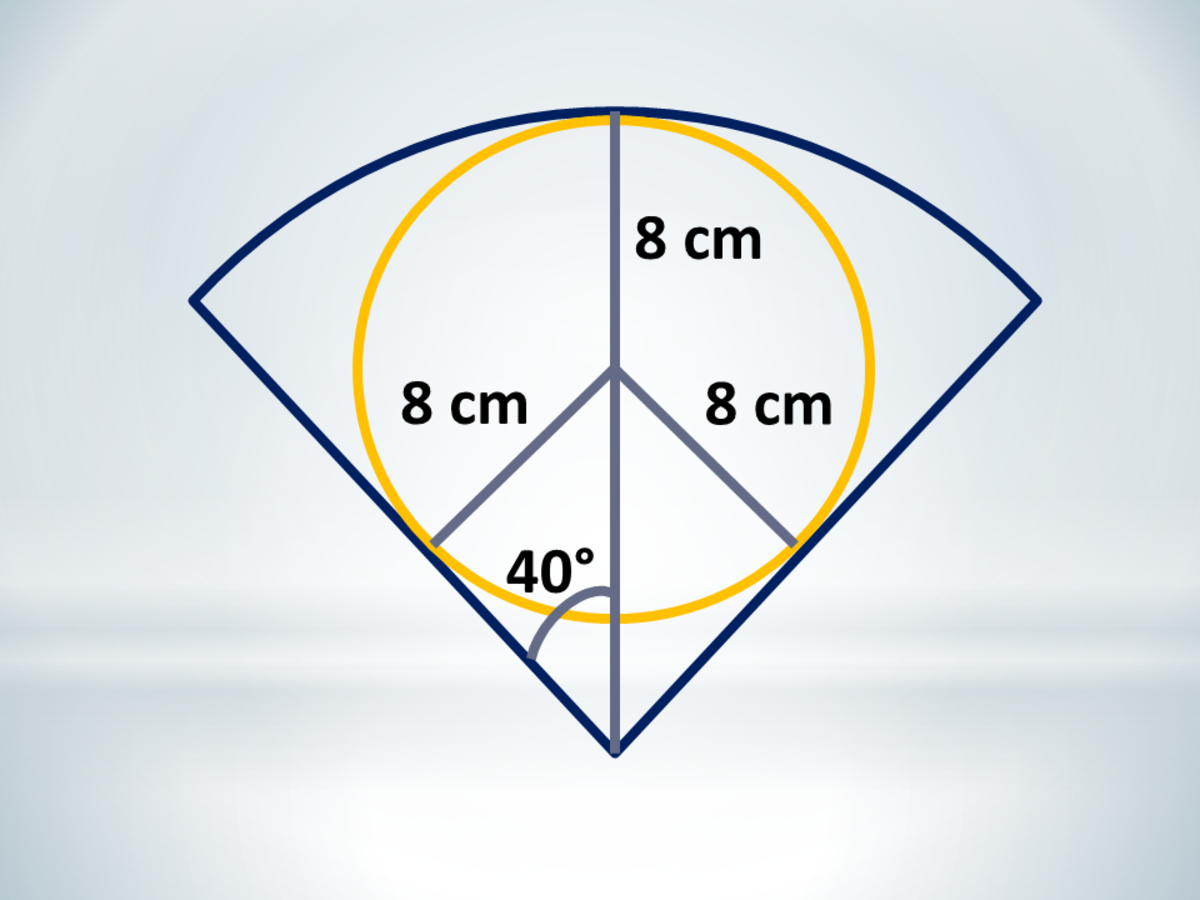Circle Inscribed in a Sector: Calculator Techniques for Circles and Triangles in Plane GeometryJohn Ray Cuevas

Calculator Technique

a. Solve for the radius of the sector.

b. Solve for the area of the sector.

c. Go to radian mode. Once in radian mode, enter Shift Answer and choose the degree sign.

Final Answer: The area of the sector is 291.83 square centimeters.

### Problem 7: Bisector of a Triangle

Given a triangle ABC with sides AB = 30 centimeters, BC = 36 centimeters and AC = 48 centimeters. Find the distance of the point of intersection of perpendicular bisectors to side BC.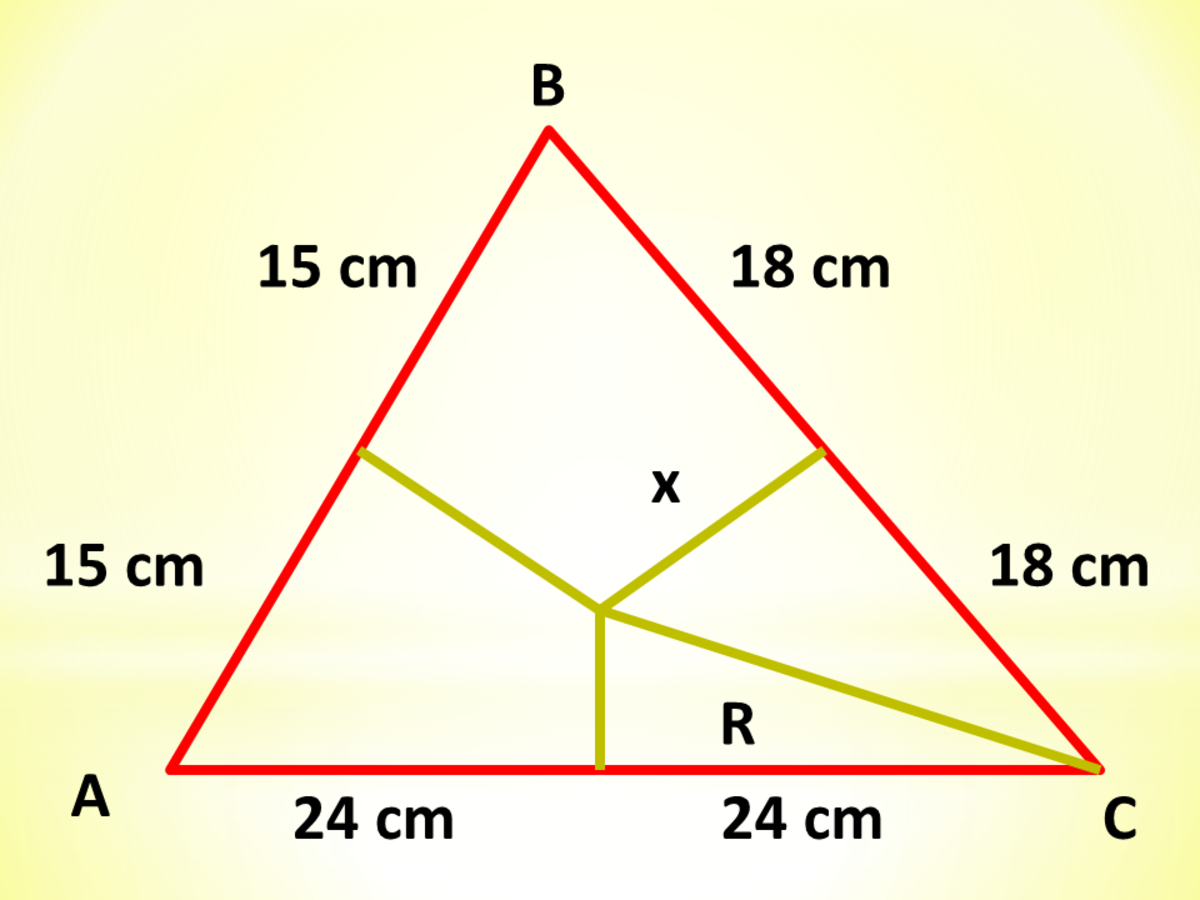Bisector of a Triangle: Calculator Techniques for Circles and Triangles in Plane GeometryJohn Ray Cuevas

Calculator Technique

a. The point of intersection of the perpendicular bisectors is the radius of the circumscribed circle. Solve for the area of the triangle using Heron's Formula.

b. Solve for the radius of the circumscribed circle.

c. Using the Pythagorean theorem, solve for the missing value x.

Final Answer: The distance from the point of intersection of perpendicular bisectors to side BC is 15.92 centimeters.

### Problem 8: Measure of Sides of a Triangle

The perimeter of a triangle ABC is 400 centimeters. If angle A is 30 degrees and angle B is 58 degrees, find the measure of side AC.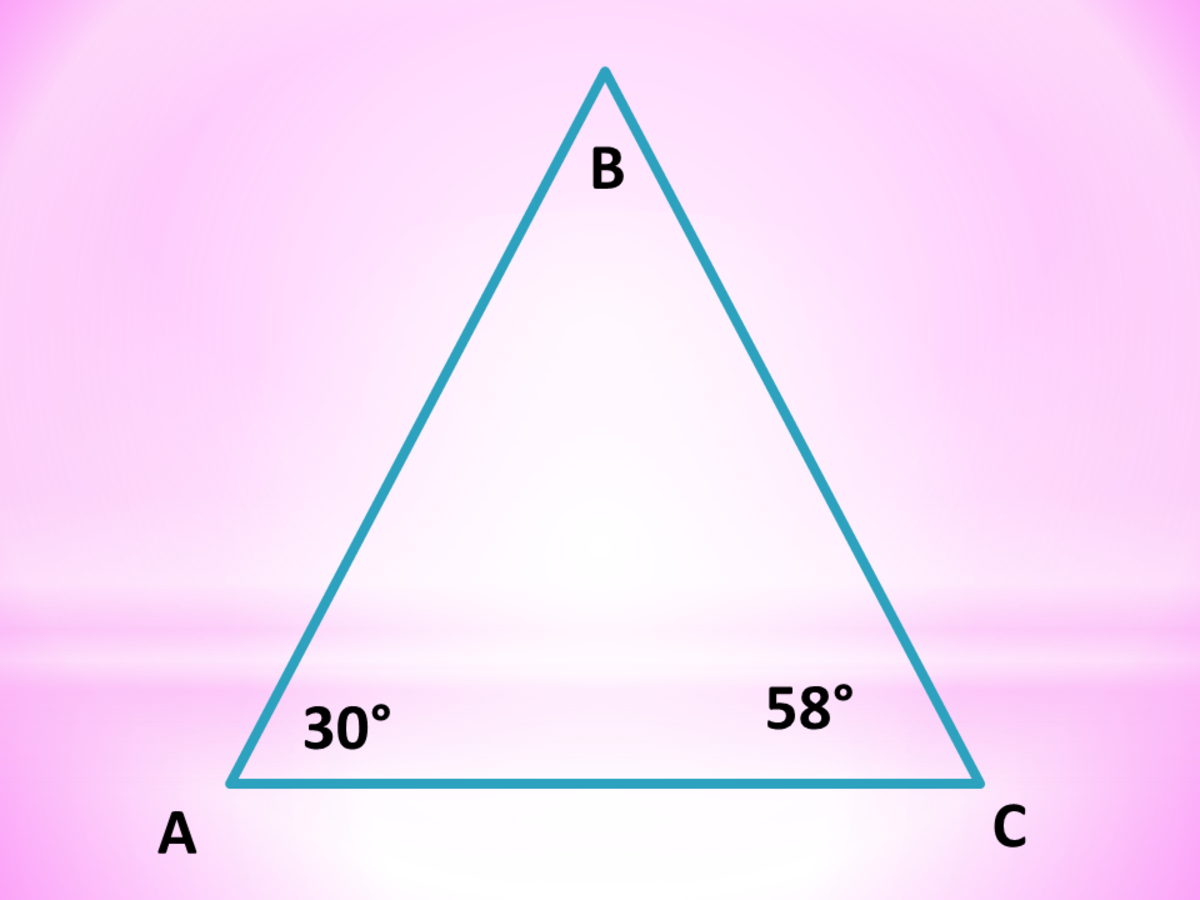Calculator Technique for Circles and Triangles in Plane GeometryJohn Ray Cuevas

Calculator Technique

a. Assume that AC = 1 then use sine law technique in solving for the sides AB and BC. Set your calculator in equation mode and input the following values.

Calculator Technique in Equation Mode

XYC

cos (30)

cos (58)

1

sin (30)

- sin (58)

0

b. Solve for the length of side AC.

Final Answer: The length of side AC is 170.31 centimeters.

### Problem 9: Chord of a Circle

A circle giving an area of 1018 square centimeters is cut into two segments by a chord 8 centimeters from the center. Find the ratio of the area of the smaller part to the larger one?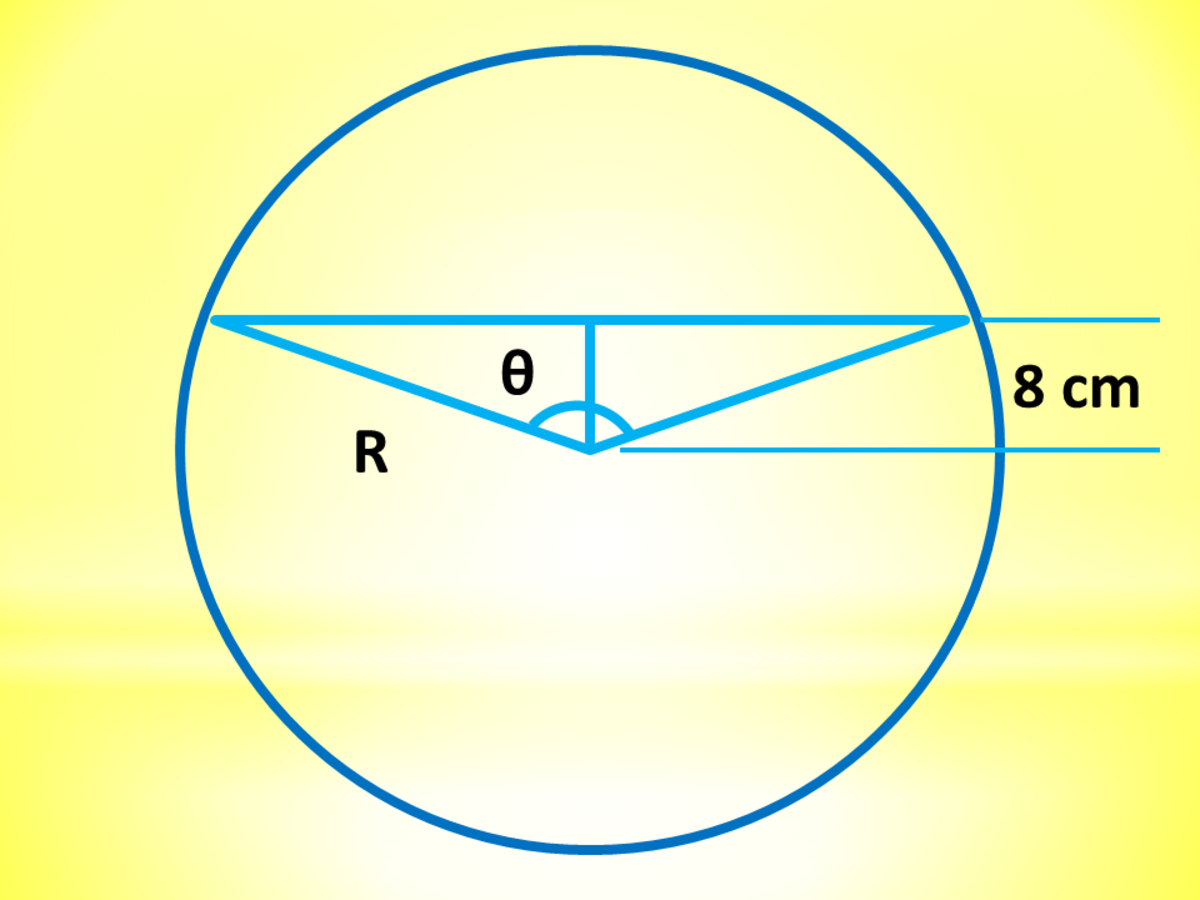Chord of a Circle: Calculator Techniques for Circles and Triangles in Plane GeometryJohn Ray Cuevas

Calculator Technique

a. Solve for the radius of the circle.

b. Solve for θ.

c. Find the area of the small segment.

d. Solve for the larger segment.

e. Solve for the ratio between the two segments.

Final Answer: The ratio between the two segments is 0.293.

### Problem 10: A Point Outside a Triangle

From a point outside an equilateral triangle, the distances to the vertices are 10 centimeters, 18 centimeters, and 10 centimeters, respectively. What is the length of one side of the triangle?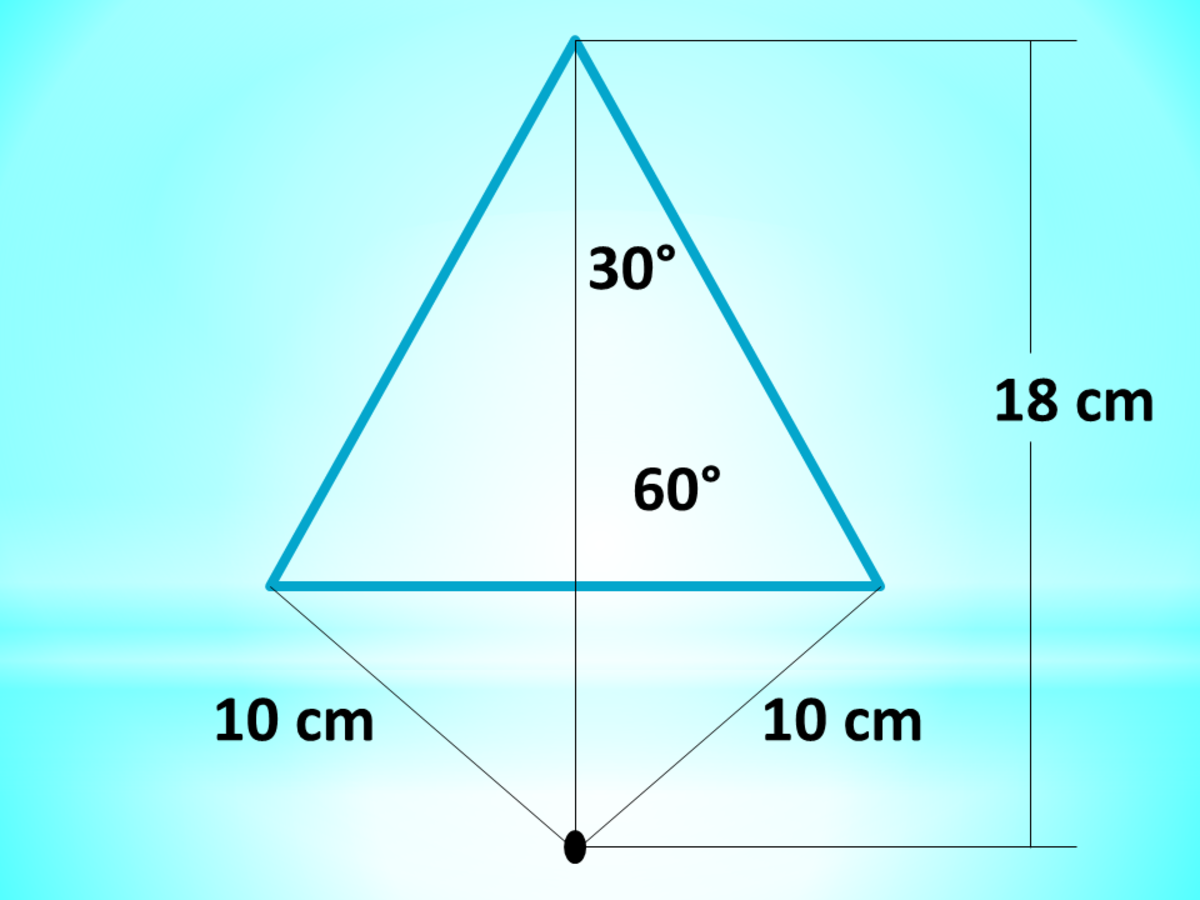A Point Outside a Triangle: Calculator Techniques for Circles and Triangles in Plane GeometryJohn Ray Cuevas

Calculator Technique

a. Solve for the length of one side X using the Cosine law.

Final answer: The length of one side of the triangle is 19.95 centimeters.

## Other Calculator Techniques

Ray (author) from Philippines on April 11, 2019:

You're very welcome, Ali Hassan. There are a lot more articles like this. You can check it in my profile. Happy hubbing!

Ali Hassan on April 11, 2019:

It's a very helpful and informative article thanks for making this

Ray (author) from Philippines on July 17, 2018:

Thanks, Syed Aabis! I appreciate it. Have a great day pal! Enjoy reading my articles.

Syed Aabis from Pakistan on July 17, 2018:

It is very informative article i will like to read more like this topic